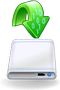•• Restorer Ultimate Documentation

# Data Formats and Multipliers

You may enter data in all numerical fields either in sectors or in bytes. If there is no letters are after the number, Restorer Ultimate assumes the numbers are in bytes.

Decimal numbers are entered as they are: 2372354

Hexadecimal numbers are entered as 0x23Fa67 or 23Fa67 hex .

The following case-insensitive notation is possible:

 b 1 byte kb 1 kb = 2^10=1024 bytes mb 1 mb = 2^20=104857 bytes gb 1 gb = 2^30=1073741824 bytes tb 1 tb = 2^40=1099511627776 bytes eb 1 eb = 2^50=1125899906842624 bytes hex A hexadecimal number sec (sector) A number is in sectors

You may also select the multipliers in the drop-down boxes .

When the multiplier has been changed, the data value will be changed according to the specific contextual commands for that field:

Suppose, the initial value is 1 GB, and the sector size of the object is 512 byte. The results will be the following:

### Command: No recalculate

Change from GB to MB: 1

Change from GB to Sectors: 1

### Command: Always recalculate

Change from GB to MB: 1024

Change from GB to Sectors: 2097152

### Command: Units type recalculate

Change from GB to MB: 1

Change from GB to Sectors: 2097152